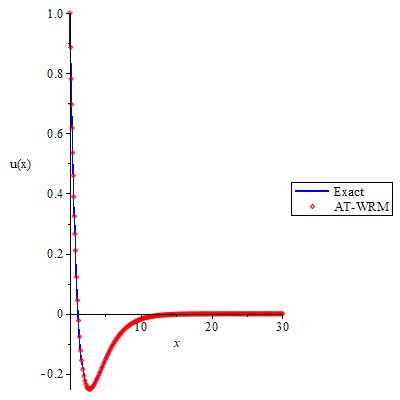# An Integral Transform-Weighted Residual Method for Solving Second Order Linear Boundary Value Differential Equations with Semi-Infinite Domain

## Authors

• E. I. Akinola Mathematics Programme, Bowen University, Iwo Osun State, Nigeria.
• R. A. Oderinu Department of Pure and Applied Mathematics, Ladoke Akintola University, Ogbomoso. Oyo State. Nigeria
• S. Alao Department of Pure and Applied Mathematics, Ladoke Akintola University, Ogbomoso. Oyo State. Nigeria
• O. E. Opaleye Department of Pure and Applied Mathematics, Ladoke Akintola University, Ogbomoso. Oyo State. Nigeria

## Keywords:

Aboodh Transform, Weighted Residual, Partition Method, Boundary Value Problem, Semi-Infinite Domain

## Abstract

Solving boundary value problem with semi-infinite domain in a conventional way or using some of the available approximate methods poses a lot of challenges or better still seems almost impossible over the years, and some of the alternative ways for crossing this hurdle is by fixing value for infinity that is in the domain. Here in this work, we present an Integral Transform (Aboodh Transform) - Weighted Residual Based Method (AT-WRM) to address the afore-mentioned challenge. Aboodh Transform was used to transform and at the same time used to find the inverse of the given differential equations while Weighted Residual via Collocation Method was used in order to avoid fixing value for infinity as usual by introducing e-ix in trial function to decay the infinity that was part of the boundary condition. The accuracy of the presented method was authenticated by solving three different problems. The excellent results obtained from the three solved problems validate the accuracy and effectiveness of the method

Dimensions

R. A. Oderinu & Y. Aregbesola, “Weighted Residual Method In A Semi Infinite Domain Using an Un-Patitioned Method”, International Journal of Applied Mathematics 25 (2012) 25.

S. Odejide & Y. Aregbesola, “Applications of method of weighted residuals to problems with semi-finite domain”, Rom. Journ. Phys. 56 (2011) 14.

M.A.Noor & S.T. Mohyud-Din, “Modified variational iteration method for a boundary layer problem in unbounded domain”, International Journal of Nonlinear Science 7 (2009) 426.

A.Adewumia, et al., “Laplace-weighted residual method for problems with semi-infinite domain”, Journal of Modern Methods in Numerical Mathematics 7 (2016) 59.

H.A.Peker, O. Karaolu & G. Oturanç, “The differential transformation method and Pade approximant for a form of Blasius equation”, Mathematical and Computational Applications 16 (2011) 507.

M. Sajid, N. Ali, & T. Javed, “A Hybrid Variational Iteration Method for Blasius Equation”, Applications and Applied Mathematics: An International Journal (AAM), 10 (2015) 15.

E.Akinola, et al., “On the Application of Sumudu Transform Series Decomposition Method and Oscillation Equations”, Asian J. Mathematics, 2 (2017) 1.

S. Khuri, “On the decomposition method for the approximate solution of nonlinear ordinary differential equations”, International Journal of Mathematical Education in Science and Technology 32 (2001) 525.

S.Abbasbandy, & T. Hayat, “Solution of the MHD FalknerSkan flow by Hankel Pade method”, Physics Letters A 373 (2009) 731.

A.M. Wazwaz, “The variational iteration method for solving two forms of Blasius equation on a half-infinite domain”, Applied Mathematics and Computation 188 (2007) 485.

S. Alao, R. A. Oderinu, F. O. Akinpelu & E. I. Akinola, “Homotopy Analysis Decomposition Method for the Solution of Viscous Boundary Layer Flow Due to a Moving Sheet”, Journal of Advances in Mathematics and Computer Science 32 (2019) 1.

A.W. Ogunsola, R.A. Oderinu, M. Taiwo & J.A. Owolabi, ”Application of Laplace Decomposition Method to Boundary Value Equation in a Semi-Infinite Domain”, International Journal of Difference Equations (IJDE) 17 (2022) 75.

M.A. Ramadan, T. Radwan, M.A. Nassar & M.A. Abd El Salam, “A Comparison Study of Numerical Techniques for Solving Ordinary Differential Equations Defined on a Semi-Infinite Domain Using Rational Chebyshev Functions by Ramadan”, Journal of Function Spaces 2021 (2021) 12 .

F. O. Obarhua & O. J. Adegboro, “An order four continuous numerical method for solving general second order ordinary differential equations”, J. Nig. Soc. Phys. Sci. 3 (2021) 42.

J.O. Kuboye, O. R. Elusakin & O.F. Quadri, “Numerical Algorithms for Direct Solution of Fourth Order Ordinary Differential Equations”, J. Nig. Soc. Phys. Sci., 2 (2020) 218.

K. S. Aboodh, “The New Integral Transform ’Aboodh Transform”, Global Journal of Pure and Applied Mathematics 9 (2013) 35.

K. S. Aboodh, “Application of new transform Aboodh transform to partial differential equations”, Global Journal of Pure and Applied Mathematics, 10 (2014) 249.

S.H. Crandall, “Engineering analysis: A survey of numerical procedures”, McGraw-Hill, 1956.

B. Finlayson & L. E. Scriven, “The method of weighted residual; a review”, Appl. Mech. Rev. 19 (1966) 735.

R. Vichnevetsky, “Use of functional approximation methods in the computer solution of initial value partial differential equation problems”, IEEE Transactions on Computers 100 (1969) 499.

R. A.Oderinu & A. S.Aregbesola, “Using Laguerres Quadrature in Weighted Residual Method for Problems with Semi Infinite Domain”, International Journal of pure and Applied Mathematics 75 (2012) 371.2022-11-11

## How to Cite

An Integral Transform-Weighted Residual Method for Solving Second Order Linear Boundary Value Differential Equations with Semi-Infinite Domain. (2022). Journal of the Nigerian Society of Physical Sciences, 4(4), 867. https://doi.org/10.46481/jnsps.2022.867

## Section

Original Research

## How to Cite

An Integral Transform-Weighted Residual Method for Solving Second Order Linear Boundary Value Differential Equations with Semi-Infinite Domain. (2022). Journal of the Nigerian Society of Physical Sciences, 4(4), 867. https://doi.org/10.46481/jnsps.2022.867